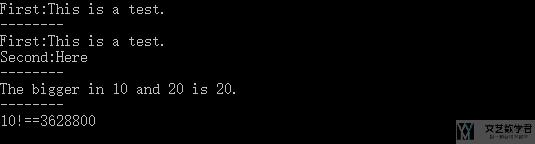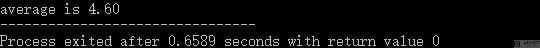# C学习笔记 – 函数

• A+

## 内容简介

• 函数的定义(注意需要函数声明)：这里我们需要注意函数使用前需要先声明
• 函数的嵌套使用：这个没什么好说的，看看就能明白；
• 递归函数：会举一个阶乘的例子进行说明；
• 数组元素作为函数实参：我们举一个求平均数的例子；

## 函数定义，嵌套，递归

1. #include<stdio.h>
2. #include<stdlib.h>
3. /*
4.     知识点
5.     - 函数的定义(注意需要函数声明)
6.     - 函数的嵌套使用
7.     - 递归函数
8. */
9. //函数的声明
10. void printTest();
11. void printTwoTest();
12. int max(int x, int y);
13. int fac(int n);
14. void printTest(){
15.     // 这里的函数声明表示没有返回值,即返回值是void类型
16.     // 这里定义的函数是无参函数
17.     printf("First:This is a test.\n");
18. }
19. void printTwoTest(){
20.     // --------
21.     // 函数嵌套
22.     // --------
23.     void printTest(); //函数嵌套也需要首先声明嵌套的函数
24.     printTest();
25.     printf("Second:Here\n");
26. }
27. int max(int x, int y){
28.     // 定义有参数的函数
29.     int z;
30.     z = (x>y)?x:y;
31.     return (z);
32. }
33. int fac(int n){
34.     // ------------
35.     // 定义递归函数
36.     // ------------
37.     int f;
38.     if(n==0||n==1){
39.         f=1;
40.     }
41.     else{
42.         f=n*fac(n-1);
43.     }
44.     return f;
45. }
46. int main(){
47.     printTest(); // 调用printTest函数
48.     printf("--------\n");
49.     printTwoTest();
50.     printf("--------\n");
51.     int a =10,b=20,c;
52.     c = max(a,b);
53.     printf("The bigger in %d and %d is %d.\n",a,b,c);
54.     printf("--------\n");
55.     printf("%d!==%d\n",10,fac(10));
56.     return 0;
57. }## 函数实参传数组

1. #include<stdio.h>
2. #include<stdlib.h>
3. /*
4.     数组元素作为函数实参
5.     - 使用数组名作为实参时,向形参传递的是数组元素的首地址
6. */
7. float average(float array[]){
8.     // 定义一个求平均数的函数
9.     int i;
10.     float sum;
11.     for(i=0;i<5;i++){
12.         sum=sum+array[i];
13.     }
14.     return sum/5;
15. }
16. int main(){
17.     float average(float array[]); // 函数的声明
18.     float score={10,2,3,1,7};
19.     printf("average is %0.2f",average(score));
20.     return 0;
21. }• 微信公众号
• 关注微信公众号
•• QQ群
• 我们的QQ群号
•